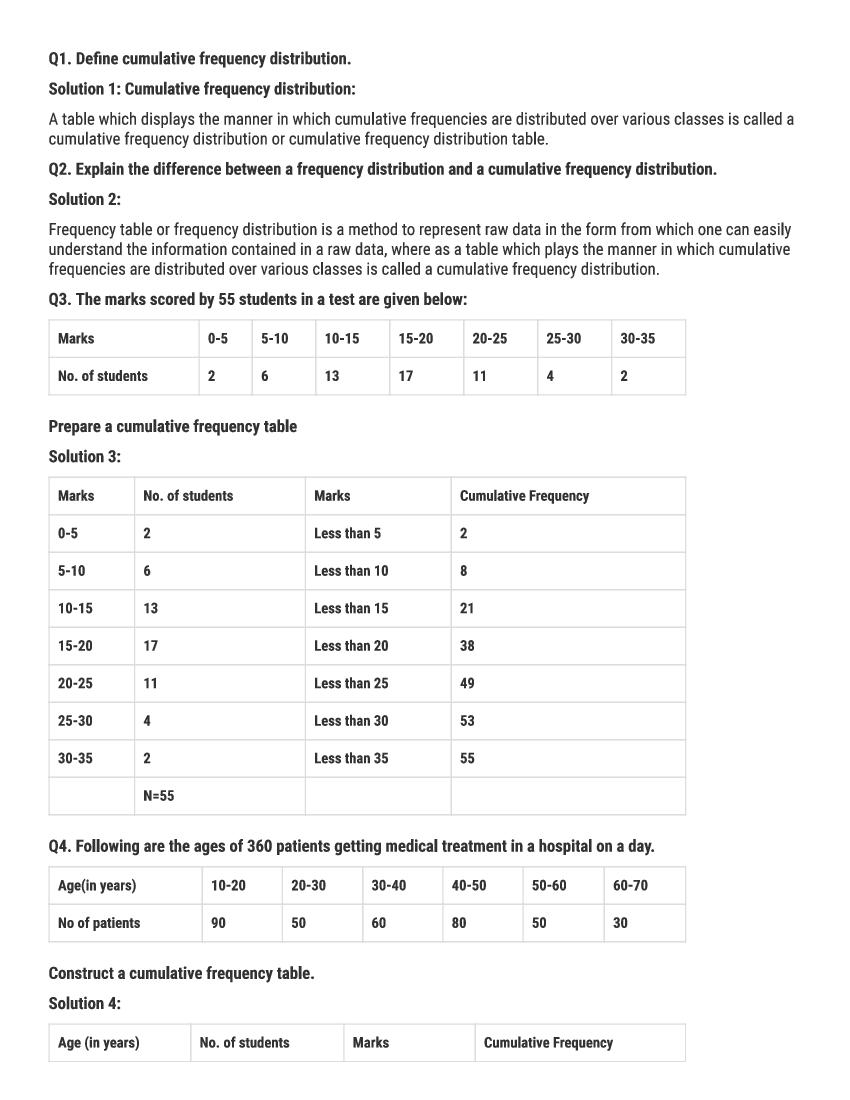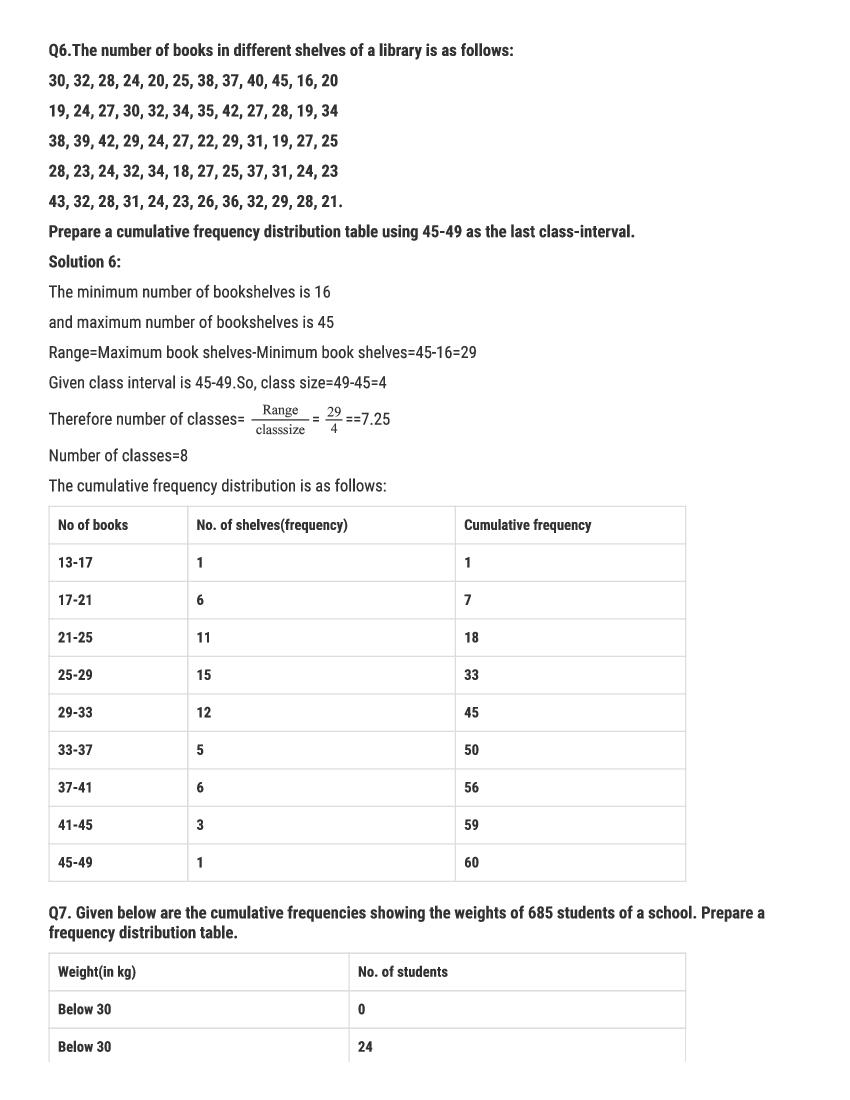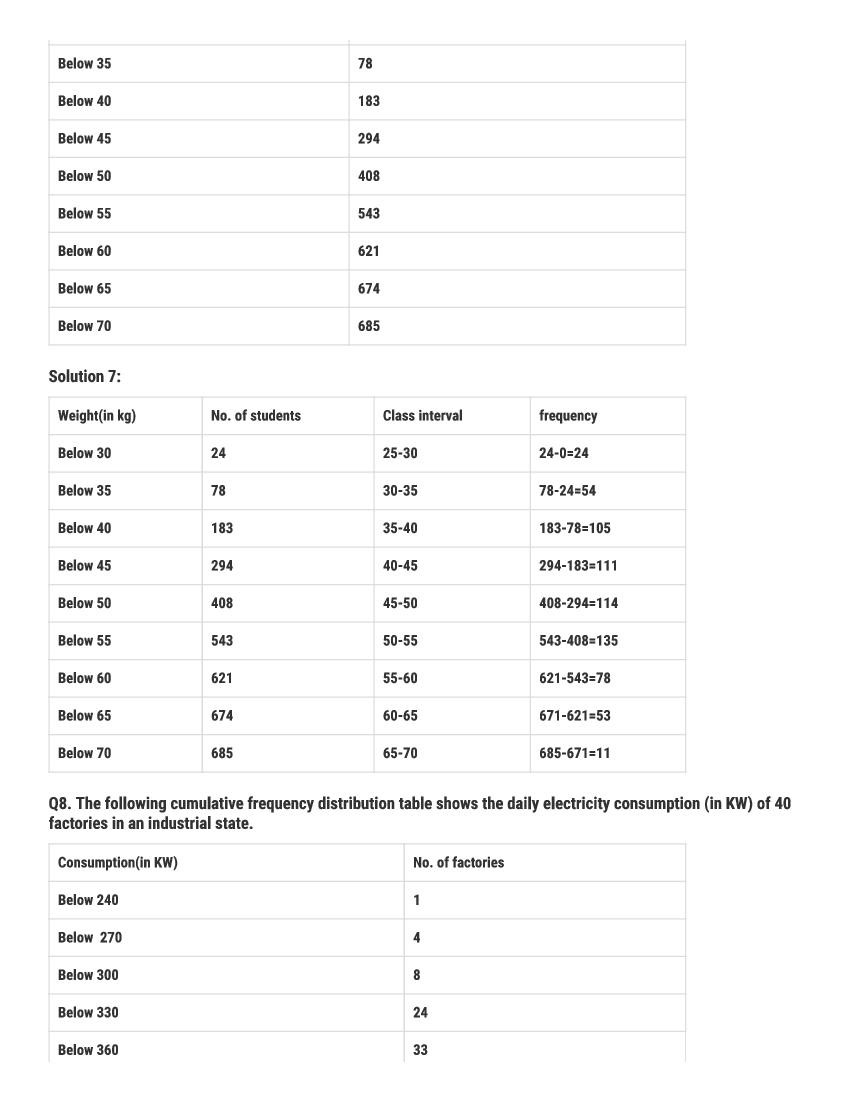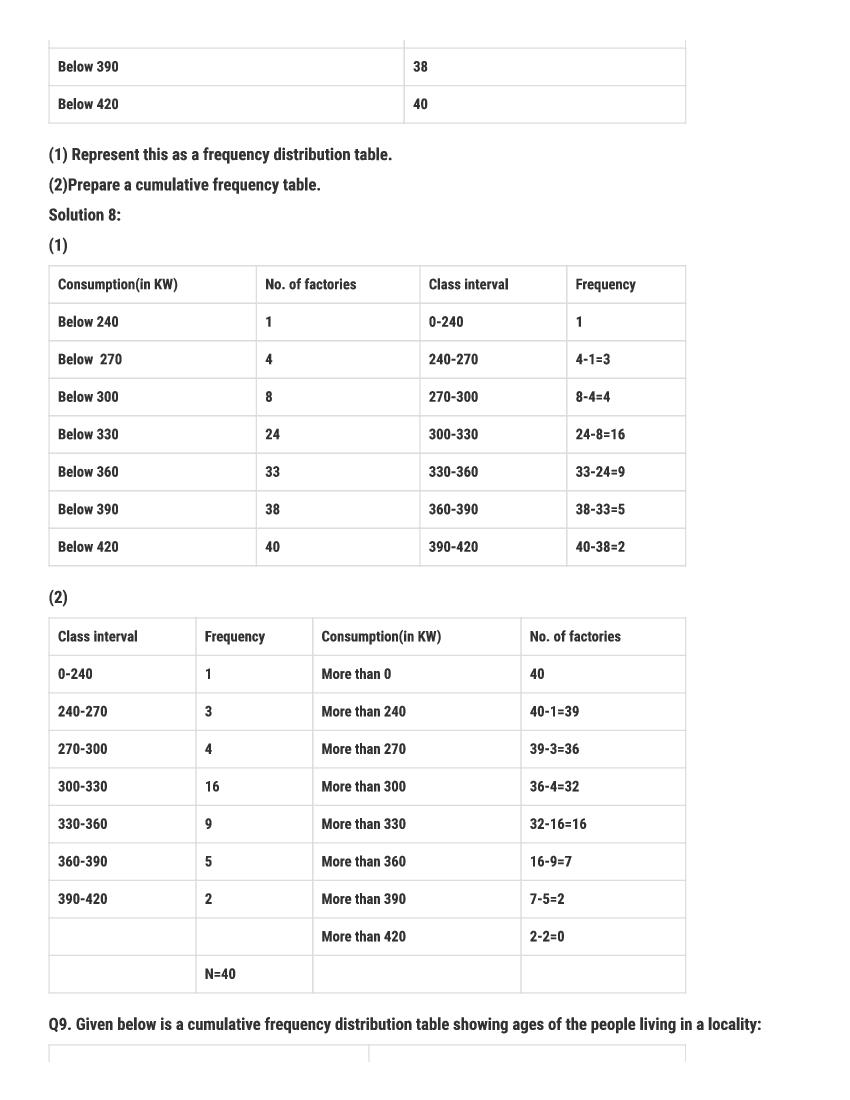# RD Sharma Solutions Class 9 Chapter 22 Tabular Representation of Statistical Data Exercise 22.2

Here you can get free RD Sharma Solutions for Class 9 Maths Chapter 22 Tabular Representation of Statistical Data Exercise 22.2. All RD Sharma Book Solutions are given here exercise wise for the chapter Tabular Representation of Statistical Data. RD Sharma Solutions are helpful in the preparation of several school level, graduate and undergraduate level competitive exams. Practicing questions from RD Sharma Mathematics Solutions for Class 9 Chapter 22 Tabular Representation of Statistical Data is proven to enhance your math skills.

 Class: Class 9th Chapter: Chapter 22 Exercise: Exercise 22.2 Name: Tabular Representation of Statistical Data

## RD Sharma Solutions Class 9 Chapter 22 Tabular Representation of Statistical Data Exercise 22.2

RD Sharma Class 9 Solutions Chapter 22 for Tabular Representation of Statistical Data Exercise 22.2 are given below.

RD Sharma Solutions Class 9 Chapter 22 Tabular Representation of Statistical Data Excercise 22.2RD Sharma Solutions for Class 9 Maths PDF download for Chapter 22 Tabular Representation of Statistical Data Exercise 22.2 is available here for free.

 « Previous Next »

### RD Sharma Solutions Class 9 Chapter 22 Exercise Wise

You can also see RD Sharma Solutions of all exercises of Chapter 22 – Tabular Representation of Statistical Data from here.

### RD Sharma Solutions Class 9 Chapter Wise

If you want RD Sharma solutions of any topic other than Chapter 22 Tabular Representation of Statistical Data, check it from here. Mathematics by RD Sharma has all chapters that are in your Class 9 syllabus.

Click to rate this post!
[Total: 3 Average: 5]